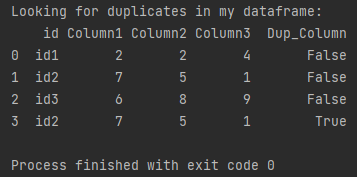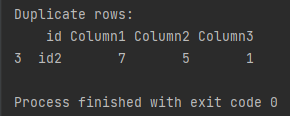Pandas How To Uncategorized How to find duplicate rows in Pandas dataframe

# How to find duplicate rows in Pandas dataframe

In this post you will learn how to find duplicate rows in Pandas dataframe.

## How to find duplicate rows

First, I’ll check if there are any duplicate rows in my dataframe. For this purpose, I will add a column in which to display information whether the row is a duplicate. I’ll use the duplicated function to check if the rows are duplicates.

```import pandas as pd

my_df = pd.DataFrame({'id':['id1','id2','id3', 'id2'],
'Column1': ['2', '7', '6', '7'],
'Column2': ['2', '5', '8', '5'],
'Column3': ['4', '1', '9', '1']})

dup_df = my_df.copy()

dup_df['Dup_Column'] = my_df.duplicated()

print(f'Looking for duplicates in my dataframe: \n{dup_df}')
```## How to list duplicate rows

You will also learn how to easily display only duplicate rows in Pandas.

In order to display the redundant rows, I will create a copy of the dataframe. Will use duplicated function.

```import pandas as pd

my_df = pd.DataFrame({'id':['id1','id2','id3', 'id2'],
'Column1': ['2', '7', '6', '7'],
'Column2': ['2', '5', '8', '5'],
'Column3': ['4', '1', '9', '1']})

dup_list = my_df[my_df.duplicated()]

print(f'Duplicate rows: \n{dup_list}')

```Pandas displays only one row which is duplicated. This is the same line we found in the first example.

## How to calculate percent changeHow to calculate percent change

In this post I show you how to calculate percent change in Pandas. You will also learn how to add a percentage change column to the dataframe.

## How to pivot tables in Pandas?How to pivot tables in Pandas?

One of the most useful features of Pandas is the ability to pivot tables, which allows you to transform data by grouping, aggregating, and reshaping it.